# AP Calculus AB : Estimating limits from graphs or tables

## Example Questions

### Example Question #1 : Estimating Limits From Graphs Or Tables

Consider the function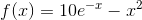.  Which Reimann sum calculation would give the best approximation of the integral fromto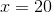?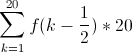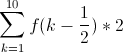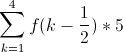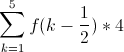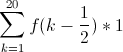Explanation:

The mid-point Reimann sum is given by this formula: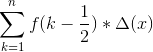, where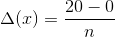, andis the number of

intervals.  Thus, if the region fromtois divided into twenty intervals,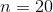and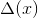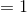.  For ten intervals,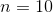and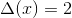.  For five internals,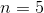and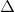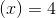.  The higher the number of intervals, the more precise the estimation.  Thus, when(and hence), the estimation is the most accurate.

### Example Question #1 : Estimating Limits From Graphs Or Tables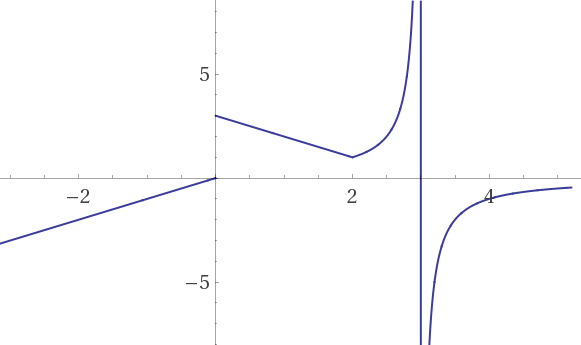What is the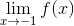?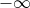Does not exist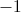Explanation:

For this value, the Limit Laws can be applied: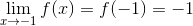### Example Question #31 : Limits Of Functions (Including One Sided Limits)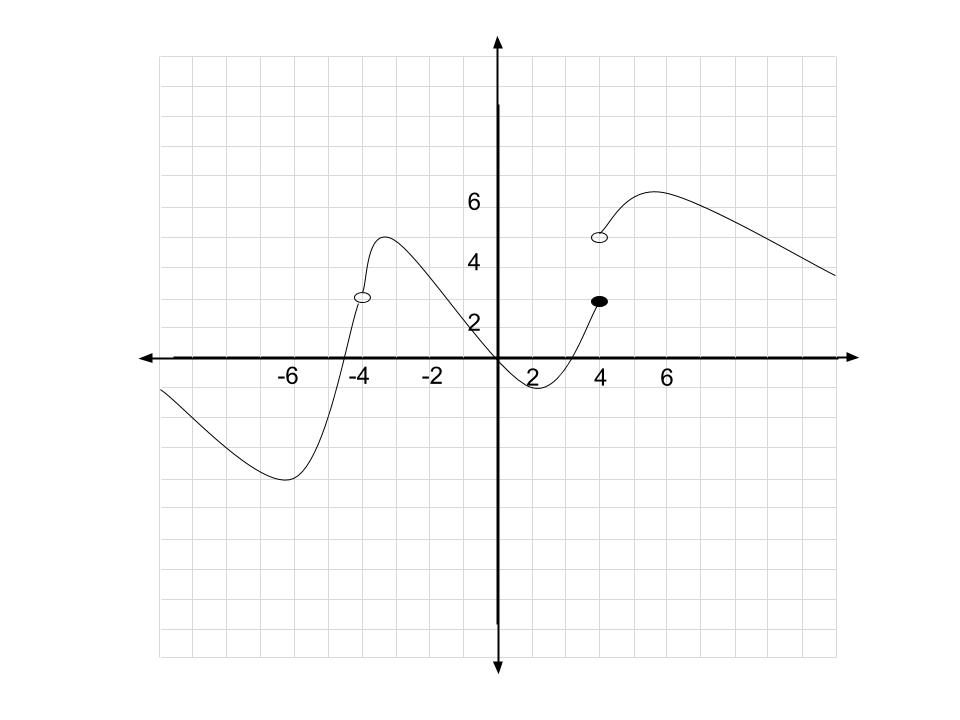True or false: In the above graph of, the value of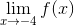is 3.

False: We can't take the limit where the function isn't defined.

False: The left and right limits exist, but neither of them is three.

False: The left and right limits exist, but exactly one of them isn't three.

True: The removable discontinuity does not affect the limit, and the right and left limits evaluate to 3.

False: Both the left and right limits are three, but the regular limit isn't.

True: The removable discontinuity does not affect the limit, and the right and left limits evaluate to 3.

Explanation:

Removable discontinuities don't affect the limiting process. The limit process is essentially saying "As you get arbitrarily close to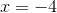, the function is getting arbitrarily close to." As you can see, whether or not the function is defined atis irrelevant, because we're want to look at values close to it, but never exactly at.

Graphically, we can see that the left and right limits are both three. If you trace the graph from either the left or right of, you will end up at.

By definition, if both the right and left limit evaluate to the same thing, the actual limit must agree. It's not possible for both of them to be three, but for the limit to disagree with them (at least in a two dimensional graph!)

Thus the correct answer is that the limit exists, and is three.

### Example Question #32 : Limits Of Functions (Including One Sided Limits)In the above graph of, evaluate the following limits: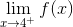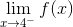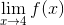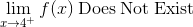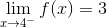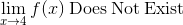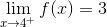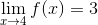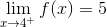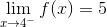Explanation:

A right limit is found graphically by starting at a point to the right of the specified x value and tracing along the graph to the left until you hit the specified x. Regardless of whether or not the function is defined, or perhaps if its defined, but not where your finger ends up, your finger should be at the limit. This is because limits don't concern themselves with what happens at the specified x value. For right limits, we're essentially asking "If I get arbitrarily close tofrom the right, what isgetting close to?"

The same holds true for left limits, but you approach them from the other side.

In this problem,is a right limit. Following the above method, we find that as we approachfrom the right, we end up at. Similarly,is a left limit. Approachingfrom the left, we see that we arrive at.

Lastly, a regular limit is defined when both the right and left limits are defined and equal. In this case, the right limit is 5 and the left limit is 3, so the regular limit does not exist